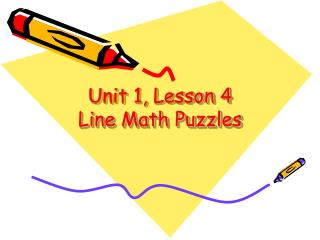DownloadDownload PresentationUnit 1, Lesson 4 Line Math Puzzles

# Unit 1, Lesson 4 Line Math Puzzles

Télécharger la présentation## Unit 1, Lesson 4 Line Math Puzzles

- - - - - - - - - - - - - - - - - - - - - - - - - - - E N D - - - - - - - - - - - - - - - - - - - - - - - - - - -
##### Presentation Transcript

1. Unit 1, Lesson 4 Line Math Puzzles

2. Goals • Develop mathematical reasoning • Practice addition facts

3. What We Will Learn Today • We are going to learn to solve line math puzzles and then you will create your own. • We will practice our addition by making groups of ten.

4. Teaching the Activity • This line math puzzle on URG pg. 51 is made of boxes connected with lines. • What is the sum of each line?

5. Teaching the Activity • What pattern do you see? • What number is in all three sums? • What other patterns do you see?

6. Line math puzzles • Each line of boxes connected by a dotted line in a line math puzzle has the same sum • Arrange the numbers 1, 2, 3, 4, and 5 on the top puzzle on Line Math Puzzles 1 activity page 15 so that each line totals 9. • Write the two number sentences on the side of the page next to the puzzles

7. Line Math Puzzles • Each line of boxes connected by a dotted line in a line math puzzle has the same sum • Arrange the numbers 5, 6, 7, 8 and 9 on the bottom puzzle on Line Math Puzzles 1 activity page 15 so that each line totals 21. • Write the two number sentences on the side of the page next to the puzzles.

8. Line Math Puzzles • When you complete DAB Pg. 15, you may do the puzzles on DAB Pg. 17. • Follow the directions for the numbers to use and the sum for each line.

9. What We Learned Today • We learn how to solve line math puzzles. • We practiced our addition by making groups of ten.

10. Extension • Make your own design for the line and box structures of the puzzle • Create line math puzzles based on a new rule: Every line has a different sum • Use 10, 11, 12, 13, 14, 15, 16, 17, 18, 19, 20 to do a line math puzzle